# polynomial with complex coefficients

The fundamental theorem of algebra - a visual proof http://weitz.de/fund/ Der Fundamentalsatz der Algebra (anschaulicher Beweis)Do not set ai=0, use checkboxes to switch on/off using coefficient ai. The function f(x) CAS (1) has probably to be set manually if cell do not actualize - mark cell + Num Eval. Hints: ai complex coefficient of p(z) - input of values polarcoordinates (mi; ωI) z=(a ; φ) polarcoordinates - sliders on top - (0<=a<=10) Zi = ai zi polynom summand in polarcoordinates =( mi ai ; ωi + i φ) -> vectors vi c(t) parametric curve of polynom p(z) - graph p(z) Pz = p(z) App - Darstellung der 3. Nullstelle p(z): :

## Find roots p(z) - z=ae^(﻿φi)

The summands of the polynomial build a vector chain (blue). A root can be found if the vector chain of the coefficients is closed - returning to the origin.
1. increase modulus (slider a) so far to have a complete free circular disc around the point of origin Z ﻿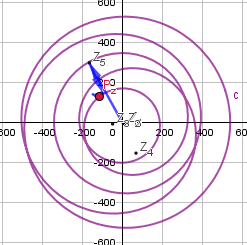2. decrease modulus to intersect graph p(z) and origin Z=(0,0) - close vector chain ﻿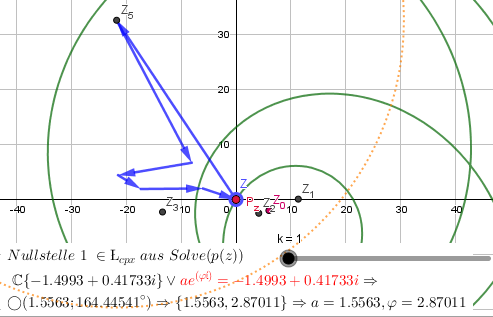3. zoom graph to position Pz (slider φ) as close as possible to the origin
4. (a ; ﻿φ ) ﻿root in polarcoordinates ==> cartesian coordinates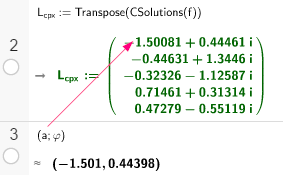5. check calculated roots in Lcpx - next intersection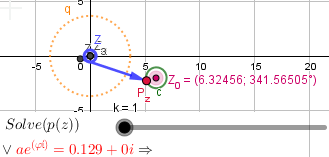## 3D Visualisation p(z)=g(x,y) Surface (open view 3D Graphics in app)Polynom surface and root points X (set property style: Level of Detail [Quality] )

## double root (real part ± imaginary part) - multiple point or repeated root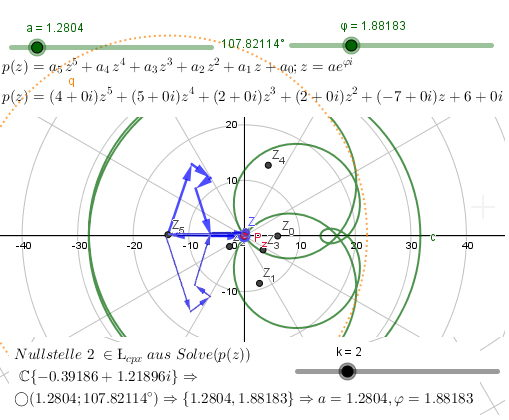f(x):=4x⁵ + 5x⁴ + 2x³ + 2x² - 7x + 6